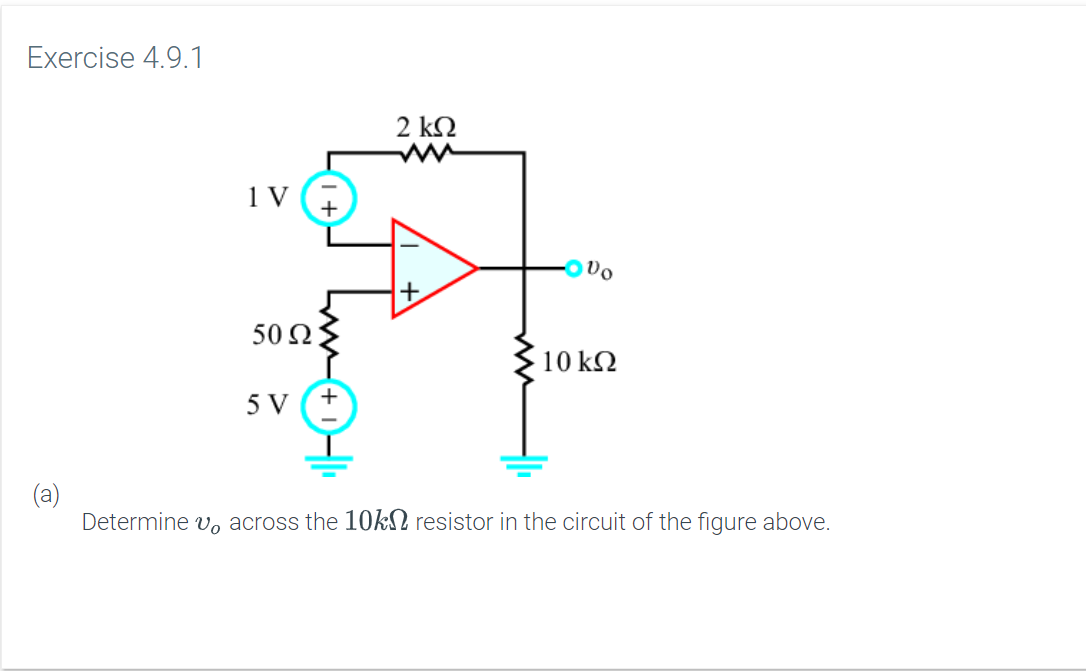Exercise 4.9.12 ΚΩODo50 Ω.10 kΩ5 VDetermine vo across the 10k2 resistor in the circuit of the figure above.

Question
Assume that even though the supply voltages are not shown, the op-amp remains in the linear region of operation.help_outlineImage TranscriptioncloseExercise 4.9.1 2 ΚΩ ODo 50 Ω. 10 kΩ 5 V Determine vo across the 10k2 resistor in the circuit of the figure above. fullscreen
Step 1

This is a diagram of voltage follower circuit, where output voltage will follow the input voltage provided. For ideal op-amp

Step 2

The voltage observed at negativ...

Want to see the full answer?

See Solution

Want to see this answer and more?

Our solutions are written by experts, many with advanced degrees, and available 24/7

See Solution
Tagged in

Electrical Engineering